# How accurate are Monte Carlo simulations

## The MONTE-CARLO experiment

The Monte Carlo experiment enables the numerical simulation of statistical populations and thus also the generation of strength values ​​for a precisely defined population of pressure vessels. This article shows as comprehensibly as possible how values ​​are generated, samples drawn and evaluated. On this basis it is also shown how the probability is determined with which samples from simulated populations pass the approval criterion “minimum bursting pressure”.Source: BAM

The Federal Institute for Materials Research and Testing (BAM) has been developing and improving statistical methods for the probabilistic safety assessment of composite pressurized gas storage facilities (see ) such as those used in particular for the storage of hydrogen (see Figure 1) for over 10 years. Aim of this among others The work presented in [2-6] is their safe operation and an approval approach that limits the design as little as possible. In order to check the effectiveness of this assessment approach, the Monte Carlo experiment has been used increasingly for the past 5 years to simulate the behavior of populations. With the help of this Monte Carlo simulation (MCS) (see also chapter 5.2.2 in ) it is possible to systematically examine acceptance criteria with regard to the different burst strengths [8,9] or load cycle strengths  of different populations . With this tool, the minimum requirements in regulations and sets of rules can be examined for their effect and also optimized.

### The principle of the Monte Carlo simulation

The Monte Carlo simulation (MCS) is based on probability and statistics. To understand the results from these simulations, it is advantageous to first build up the approach step by step with a simple and comprehensible example. The principle of the MCS can be described by an experiment to approximate the number p (“Pi”). The term “approximation of p” is understood here to mean the increasingly refined estimation of p via a geometrical consideration with the aid of statistical means.

Figure 2 shows a unit square with an enclosed unit circle.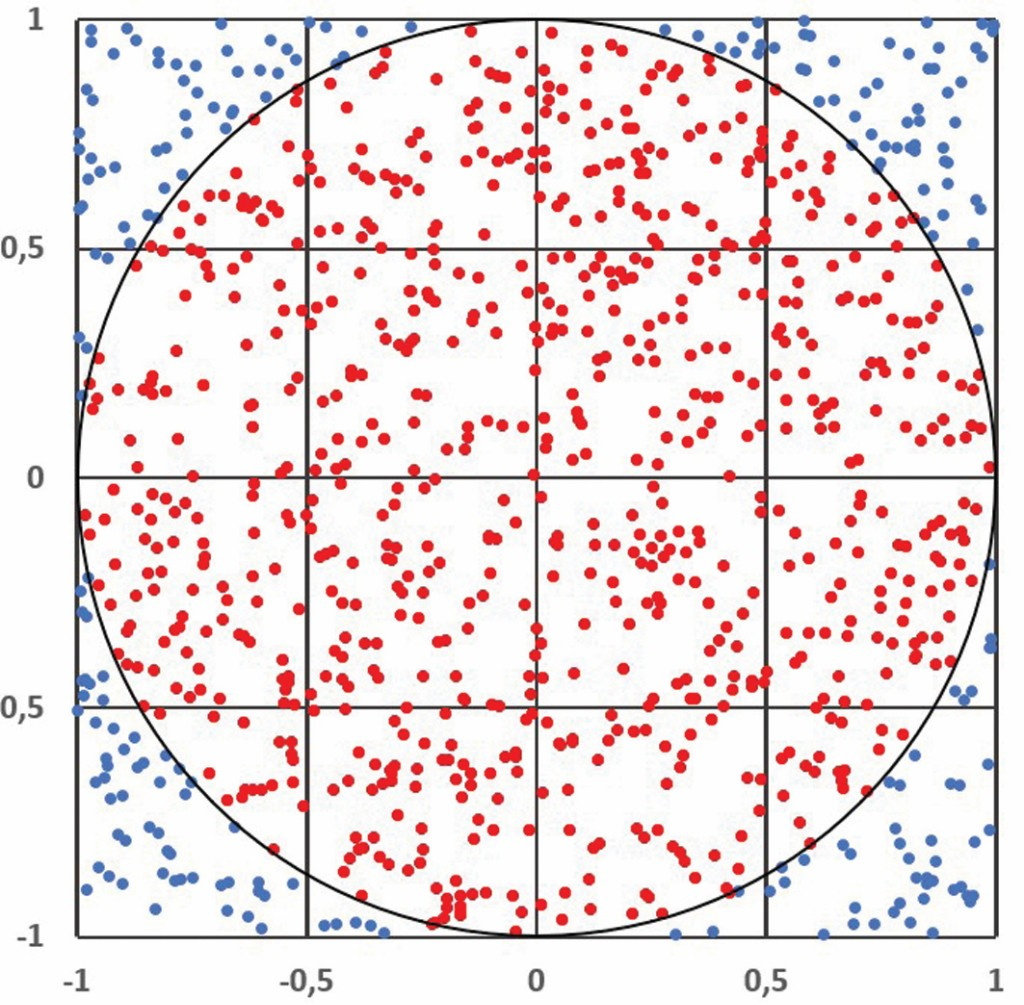Fig. 2 The MCS for approximating the number “”.

Photo: BAM

It shows points in the diagram from randomly and independently generated numerical values. It is expected that the number of generated points in the unit circle (PK) in relation to the number of points (PQ) in the unit square behaves in the same way as the ratio of the associated areas AK and AQ. That means: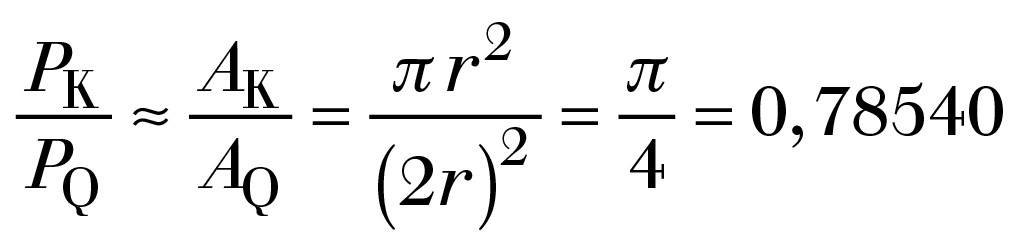For the sake of clarity, this is shown in Figure 2 using only 1,000 points. The result of this experiment depends on the number of points considered in Table 1.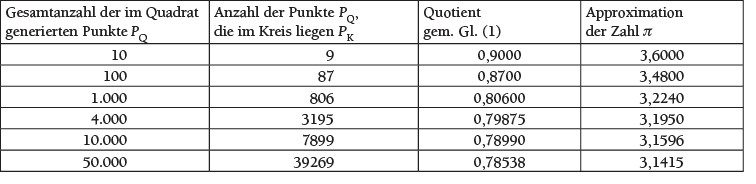Table approximation of the number pi.

The numerical results in Table 1 show how a good approximation of the number p can be statistically determined with experiments of the MCS through the random and independent arrangement of points in Figure 2. In addition, the series of numbers in the selected experiment show that the higher the number of points generated, the better the approximation. The results from the series of simulations converge to the exact solution.

### Entry into the programming of the Monte Carlo simulation based on the polar method

The MCS is based on random numbers and thus on the use of a random generator. The Polar method is shown below as an example of a possible procedure for a normally distributed MCS.

The polar method by George MARSAGLIA and Thomas A. BRAY is a possible method for generating normally distributed random numbers and can serve as a random number generator for the required basic data in the MCS. The polar method goes back to the Box-Müller algorithm for generating normally distributed random variables. This algorithm uses Euclidean coordinates.

The polar method is based on random points in the plane, which are roughly evenly distributed in an imaginary unit circle r = 1. The coordinates for the points are generated from random numbers.

The following example is carried out for a small population <100.

### Description of the sequence for generating random numbers using the polar method (see Figure 3)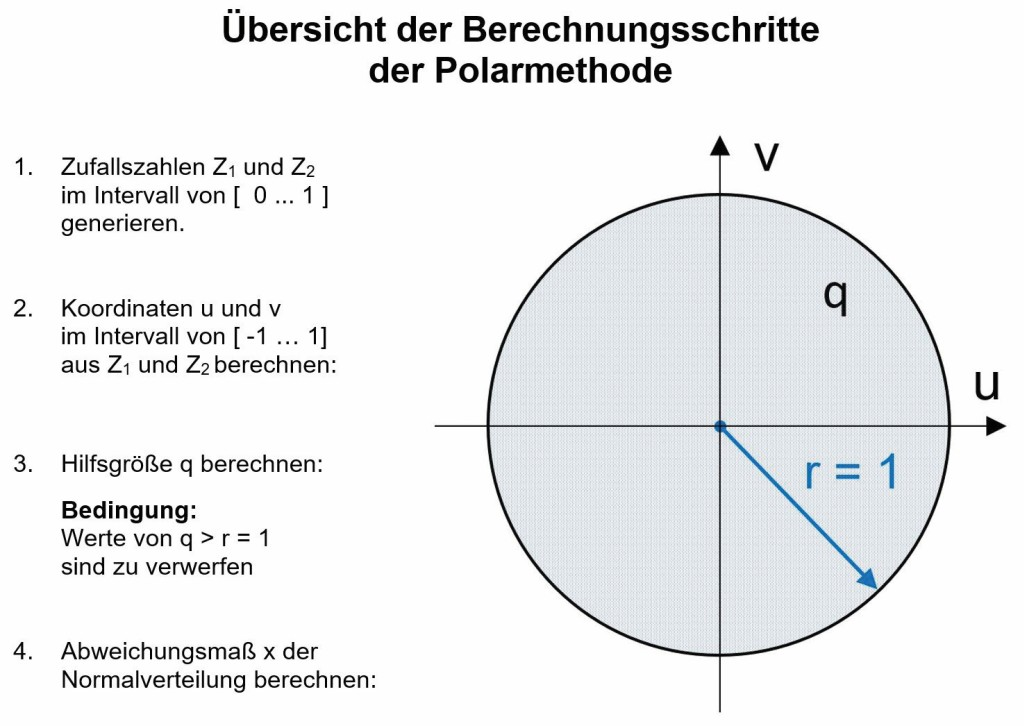Figure 3 Polar method is based on the unit circle with the radius r = 1

Photo: BAM

In the polar method, according to , there are two random numbers Z for each simulated individual1, Z2 to be generated independently by means of a random number generator in the interval from [0… 1]. The values ​​generated in this way are shown as points in the following figures.1)

In the next step, the values ​​for the coordinates in the unit circle u and v are to be transformed from the previously generated paired random numbers according to the following equations into the interval from [-1… 1].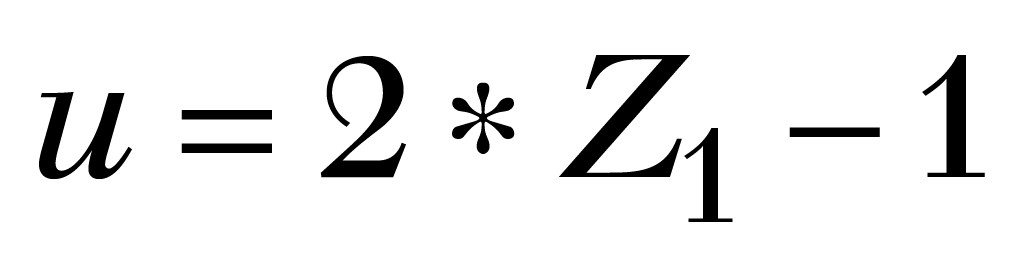In a third step, the value of the auxiliary quantity q is to be calculated for each individual i: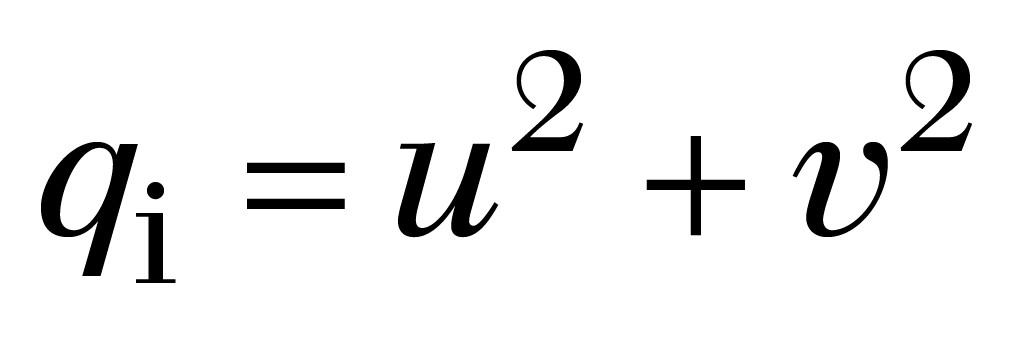If there are numerical values ​​for q for which q = r> 1, these individuals must be discarded. The results for qi are shown in Figure 4.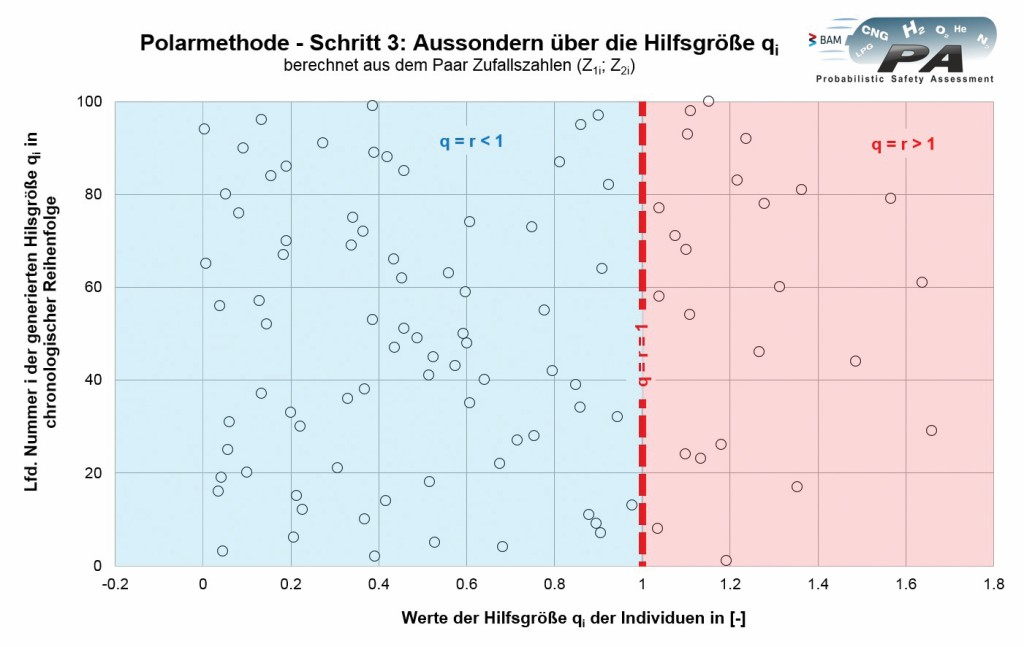Figure 4 Values ​​for the auxiliary quantity q.

Photo: BAM

In a fourth step, visible in Figure 3, the values ​​for the degree of deviation x of the normal distribution are calculated: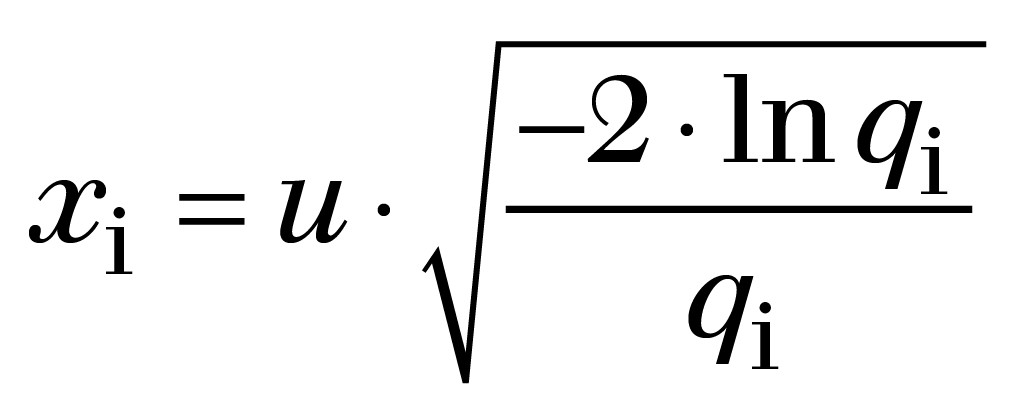This gives the values ​​shown in Figure 5. Each point stands for an individual or the considered property of the individual. The set of all points shows the entire generated population of individuals.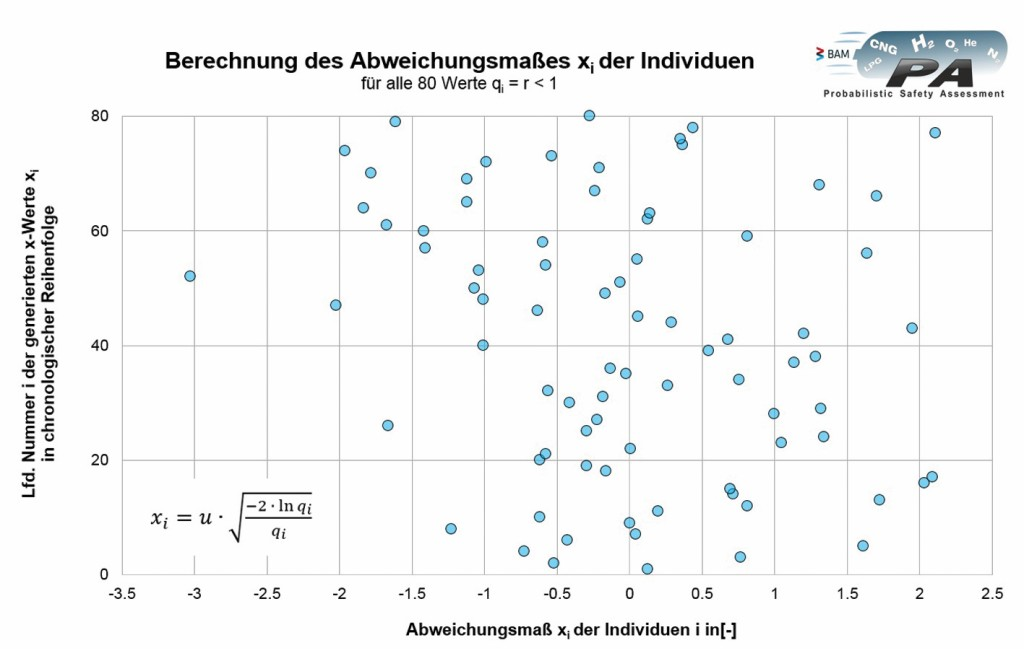Figure 5 Cloud of individuals with the deviation measure xi.

Photo: BAM

By applying the condition in the third calculation step, the numerical values ​​of q or the individuals i that lie outside the unit circle (q = r> 1) are discarded. If substitute values ​​are not regenerated, this reduces the number of simulated individuals in the further calculation of the polar method accordingly.

Normally distributed properties of individuals can not only be generated with the polar method. In the meantime, it can be assumed that common programs contain corresponding generators for random numbers that automatically generate a value cloud as shown in Figure 5.

### Generation of populations using the Monte Carlo simulation

In the following, the generated random numbers are used to simulate the “bursting strength” property of a generated population of pressure vessels made of composite materials (composite storage tanks), such as those used for storing hydrogen in vehicles or on trailers.

Here, the properties of the population are determined by the parameter “mean value” of all bursting strengths Wμ and the “standard deviation” of these bursting strengths Ws described. More precisely, these central properties W of the total population, as well as the properties of random samples used in a comparable manner below, are related to the maximum operating pressure. This normalization results in a universal numerical value that can be easily represented and evaluated in a standardized working diagram specially prepared for this purpose according to Chapter 3.1 from .

In order to achieve the bursting strength WBi of an individual i, the generated deviation measure of an individual is combined with the assumed properties of the total population (see Chapter 3.4 from ) (Fig. 6):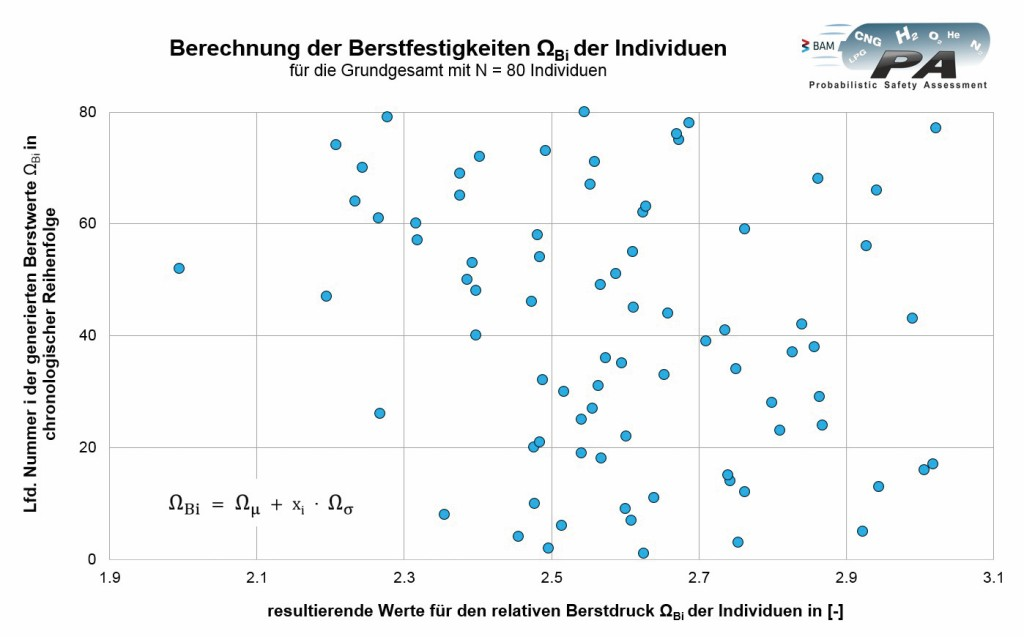Fig. 6 Values ​​for the relative burst pressure BI.

Photo: BAMThe following example is based on the following specifications for the population:

W.μ= 2.6 for the “mean burst pressure” and

W.s= 0.2 for the "standard deviation"

Checking the statistical distribution of the generated individual values ​​for burst strength

In principle, it is always useful to check whether the assumed distribution (here GAUSSian normal distribution2)) was actually generated (see ). A simple test not only shows the quality of the values, but also whether a "technical" error was made in the generation.

Pressure vessels are designed and manufactured for a guaranteed minimum burst pressure. In fact, the real burst pressures fluctuate considerably, so that it can never be ruled out that the guaranteed minimum burst value will not be exceeded by a few individuals.

To prepare the values ​​WBi for the representation in the GAUSSian probability network, please refer to Chap. 3.2.1 referenced from .

Figure 7 shows the comparison of the generated strength values ​​(points) with an ideal normal distribution (straight line).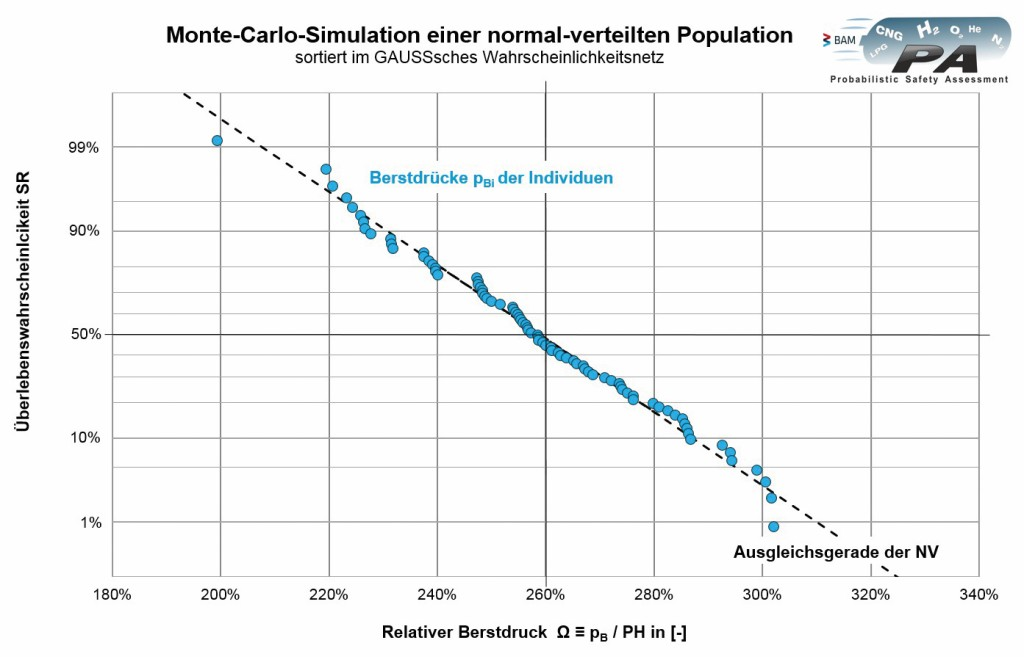Figure 7 Checking in the GAUSSian probability network (normal distribution ND).

Photo: BAM

Since all values ​​scatter closely around the straight line, there is no indication that the generated values ​​are not normally distributed or that the evaluation of the population does not match the individuals. The broken line in Figure 7 represents the best-fit straight line. The mean value of the population or total population with a thickness Q of 80 values ​​is given by the ordinate value of the best-fit straight line at 50% survival probability SR. The gradient of the regression line describes the standard deviation of the relative burst pressure of the generated population.

### Generation of samples with the Monte Carlo simulation

If you want to simulate real test results, you have to take the relevant samples from a population. This can be simulated well with the MCS. For this purpose, the specified number n of test samples is "drawn" in a random sample and statistically evaluated using a new random principle or using the randomly created chronology when generating the population. This is shown for the data example selected in Figure 8 for a sample size of n = 3.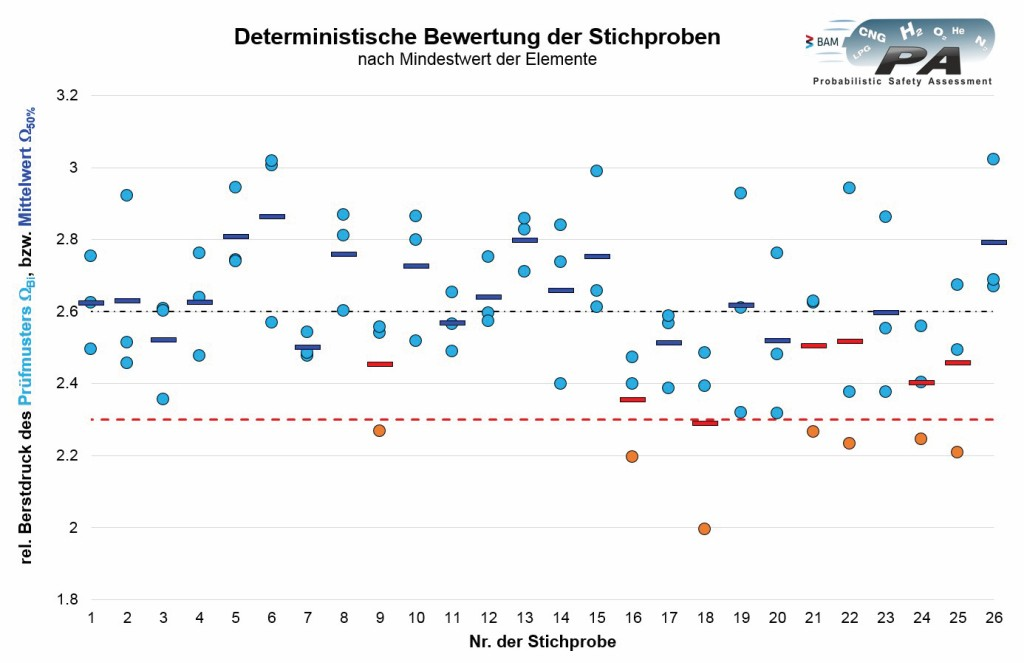Fig. 8 Evaluation of the random samples according to the individual test results.

Photo: BAM

The three individual values ​​of the samples are shown above the consecutive number of the sample.

In the next step, a classic acceptance criterion is added to the consideration. A minimum value of 2.3 times the maximum operating pressure that occurs is required for the relative burst pressure of each individual. This corresponds to a minimum value of twice the test pressure for pressure vessels according to , if the maximum operating pressure and thus the gas pressure at 65 ° C does not exceed a value of 85% of the test pressure, as is the case, for example, in FIG. B. is the case for hydrogen. All individual values ​​from the random samples that meet the minimum value are shown in blue in Figure 8 (passed), individual values ​​below the minimum value are shown in orange (failed). The associated mean values ​​of a sample are marked with a horizontal bar. The targeted overall average of 2.6 has been added. In this way, the acceptance criteria for the burst test of various regulations can also be considered .

Figure 9 shows the working diagram introduced in Section 3.1 from  to illustrate the “bursting strength” property.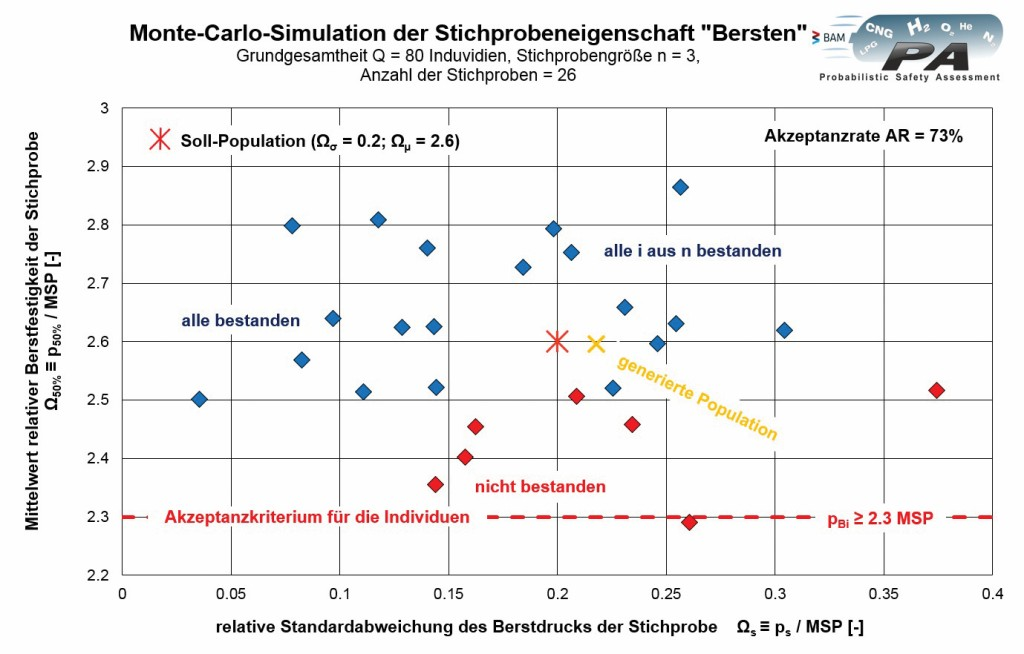Figure 9 Simulated random samples for the strength property “bursting”.

Photo: BAM

The mean value and standard deviation of the respective sample can be calculated from the summary of the values ​​of bursting properties from 3 individual containers in each sample. This means that the simulated sample properties can be represented as one point in the diagram. From the 80 individuals generated, a total of 26 samples of 3 individuals can be drawn and statistically evaluated.

If all individuals in a sample meet this deterministic minimum value according to Figure 8, the point that represents the entire sample in Figure 9 is shown in blue. If the sample contains one or more individuals who do not achieve the required strength, the sample is shown in red.

If you now put the number of blue points (19 samples with deterministically sufficient individuals) in relation to the total number of samples (26), you get the acceptance rate. This is the probability that all elements from a drawn sample will pass the test. The acceptance rate for the population simulated here (Ws = 0.2; W.μ = 2.6) is thus 73%.

If one also adds the probabilistic assessment - as shown in [6,7,9,10] - different effects of test criteria bursting  and load changes  of the corresponding regulations for the approval of prototypes of composite pressure vessels can affect their effectiveness with regard to the control of the probability of failure are examined.

Of course, according to the approach shown here with regard to distribution and scope3) different populations can be examined for different properties. The influence of the selected sample size n (= 3..7..11 ..) or the sequence in the compilation and grouping of samples can also be simulated.

### Conclusion

The Monte Carlo simulation is a suitable method to determine strength properties such as B. burst pressure, or to simulate the load cycle strength of entire populations of pressure vessels.

This simulation of pressure vessel populations and / or random samples from these populations enables analyzes that would otherwise not be possible due to their very large expenditure of time or economy.

This in turn allows the systematic analysis of the effectiveness of deterministic minimum requirements and the optimization of the corresponding approval regulations. TS713

1)For example, the "Random number ()" function can be used in MS Excel for this purpose.

2)Measured values ​​of many natural, economic and engineering processes can be described in a very good approximation by the normal distribution (NV). A series of tests at BAM have shown that the bursting strength of most types, at least when new, can be described by the NV . But there are also a few, as yet unpublished counterexamples.

3)In the applications of the MCS for the consideration of large populations of possibly many millions of individuals, it is common to use software packages geared towards statistical tasks, in contrast to the clear example shown.

literature

 Mair, G. W.; Lau, M .: Contribution to the assessment of the fatigue-related failure safety of composite pressure vessels. Technical Monitoring (TÜ) Vol. 49 (2008), No. 11/12 (Nov./Dec.), Pp. 33 - 38. ISSN 1434–9728

 Mair, G. W .; Clipper; Q .: Statistical evaluation of test results for the assessment of the residual strength of composite pressure vessels. TS 3 (2013) No. 7/8, pp. 41-49

 Mair, G. W .; Hoffmann, M .: Type examination of composite pressure vessels, probabilistic consideration of the minimum burst requirement according to the standard. TS 3 (2013) No. 11/12, pp. 48-54

 Mair, G. W .; Becker, B .: Influence of the sample size on the evaluation of the survival probability of pressure vessels made of composite materials. TS 4 (2014) No. 7/8, pp. 59-65.

 Becker, B .; Mair, G. W .: Risk and safety level of composite pressure vessels. TS 5 (2015) No. 11/12, pp. 38-44

 Mair, G. W .: Safety assessment of composite pressurized gas containers - potential of statistical methods beyond current regulations, Berlin: Springer-Verlag 2016.

 Mair, G.W .: Safety Assessment of Composite Cylinders for Gas Storage by Statistical Methods - Potential for Design Optimization Beyond Limits of Current Regulations and Standards, Springer International Publishing AG 2017

 Becker, B .; Mair, G. W .: Statistical analysis of burst requirements from regulations for composite cylinders in hydrogen transport. Materials Testing 59 (2017) 3, pp. 226-232

 Mair; G. W., et al .: Composite storage systems for compressed hydrogen - systematic improvement of regulations for more attractive storage units, International Journal of Hydrogen Energy (2018), https://doi.org/10.1016/j.ijhydene.2018.04.068.

 Mair; G. W., et al .: Monte Carlo analysis of minimum load cycle requirements for composite cylinders for hydrogen, International Journal of Hydrogen Energy (2018), https://doi.org/10.1016/j.ijhydene.2018.09.185.

 DIN ISO 5479, Statistical Evaluation of Data - Tests for Deviations from Normal Distribution.

 EN 12245 (2012) Transportable gas cylinders - fully wrapped composite cylinders. CEN: European Standard, Brussels.

From Dr.-Ing. Georg W.Mair and Dipl.-Ing. (FH) Manfred Spode

Dipl.-Ing. (FH) Manfred Spode, work area “Topic Energy - Gases”, Dept. 3 “Containers for dangerous goods”, Federal Institute for Materials Research and Testing (BAM); Berlin.
Dr.-Ing. Georg W.Mair Head of the work area “Energy - Gases”, Dept. 3 “Containers for dangerous goods”, Federal Institute for Materials Research and Testing (BAM); Berlin.